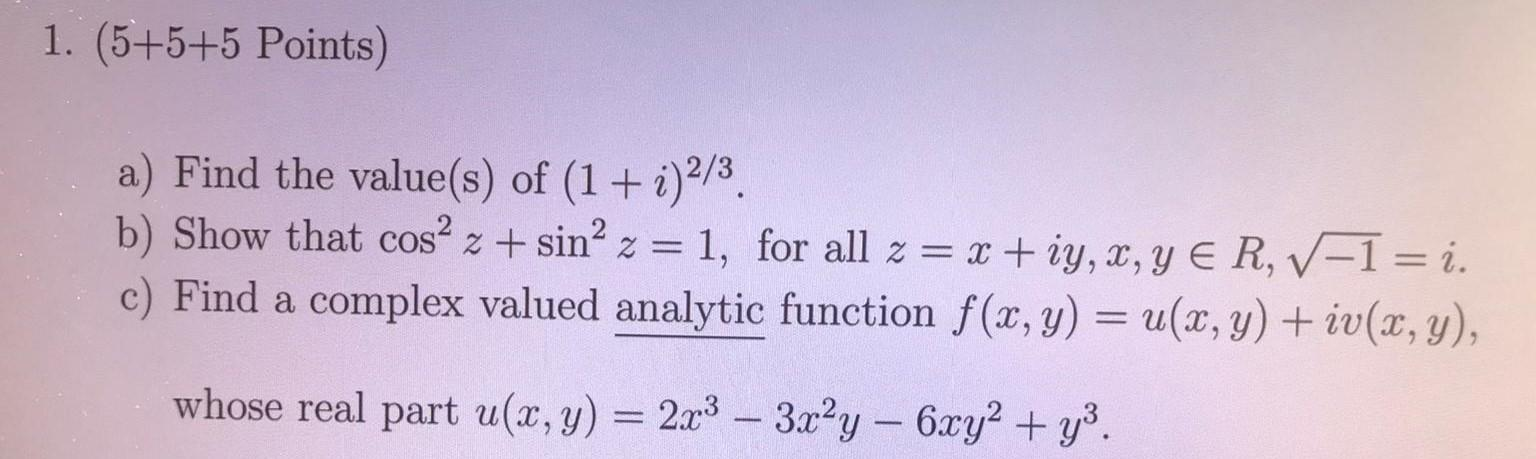### Create an Account

Home / Questions / (5+5+5 Points) a) Find the value(s) of (1 + i)2/3. b) Show that cos? 2 + sinº 2 = 1, for a...

# (5+5+5 Points) a) Find the value(s) of (1 + i)2/3. b) Show that cos? 2 + sinº 2 = 1, for all z = x +iy, x, y E RV-1 = i. c) Find a complex valued analytic function f(x, y) = u(x,y) + iv(x,y), whose re

(5+5+5 Points) a) Find the value(s) of (1 + i)2/3. b) Show that cos? 2 + sinº 2 = 1, for all z = x +iy, x, y E RV-1 = i. c) Find a complex valued analytic function f(x, y) = u(x,y) + iv(x,y), whose real part u(x, y) = 2x2 – 3x²y — 6xy2 + y).Jun 03 2021 View more View LessSubscribe To Get Solution